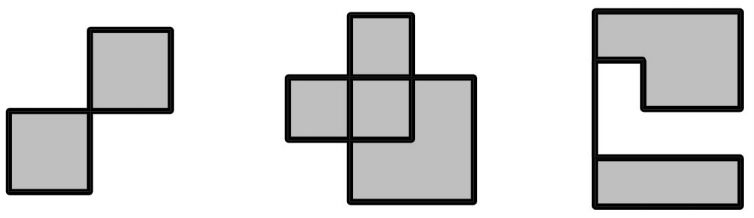시간 제한 메모리 제한 제출 정답 맞은 사람 정답 비율
5 초 1024 MB 1 1 1 100.000%

## 문제

Gary has been trying to generate simple orthogonal polygons for his geometry homework, but his algorithm seems to be having some issues. After some good hours of debugging, he finally realized what is the problem: apparently, the polygons that he was generating may contain self-intersections, which was not at all what he intended!

More specifically, the “polygons” that Gary has generated are represented by a list of $n$ points $p_i = (x_i , y_i)$, forming a closed polygonal chain. The polygonal chain may contain self-intersections. The segments formed by every two consecutive points $(x_i , y_i)$ and $(x_j , y_j )$ in the chain are either vertical or horizontal.

The polygonal chains for the example test cases are illustrated below (not to scale):You have decided to help Gary fix this issue, by computing a simple (not self-intersecting) polygon with vertical and horizontal segments that fully contains the chain, and its area is as small as possible. What is the area of such a polygon?

Formally, you have to compute the infimum of the areas of all simple orthogonal polygons that contain all the segments $[p_i , p_j ]$, for every two adjacent points $p_i$ and $p_j$.

## 입력

The first line of the input will contain a positive integer $n$ ($4 \le n \le 100\,000$). The following $n$ lines will contain the points $(x_i , y_i)$ in order ($1 \le x_i , y_i \le 10^6$). No two consecutive points coincide, and there are no two consecutive vertical segments or two consecutive horizontal segments.

## 출력

Output a single non-negative integer, the infimum of the areas of all simple polygons that enclose the closed polygonal chain. It can be proven that the answer is always integer.

## 예제 입력 1

6
1 1
6 1
6 11
11 11
11 6
1 6


## 예제 출력 1

50


## 예제 입력 2

8
2 4
2 1
4 1
4 3
1 3
1 2
3 2
3 4


## 예제 출력 2

6


## 예제 입력 3

10
1 1
1 5
4 5
4 3
2 3
2 4
1 4
1 2
4 2
4 1


## 예제 출력 3

8


## 힌트

In examples 1 and 3, there are no simple polygons with areas exactly equal to $50$ and $8$, respecively; however, there exist simple polygons with areas arbitrarily close to these values.